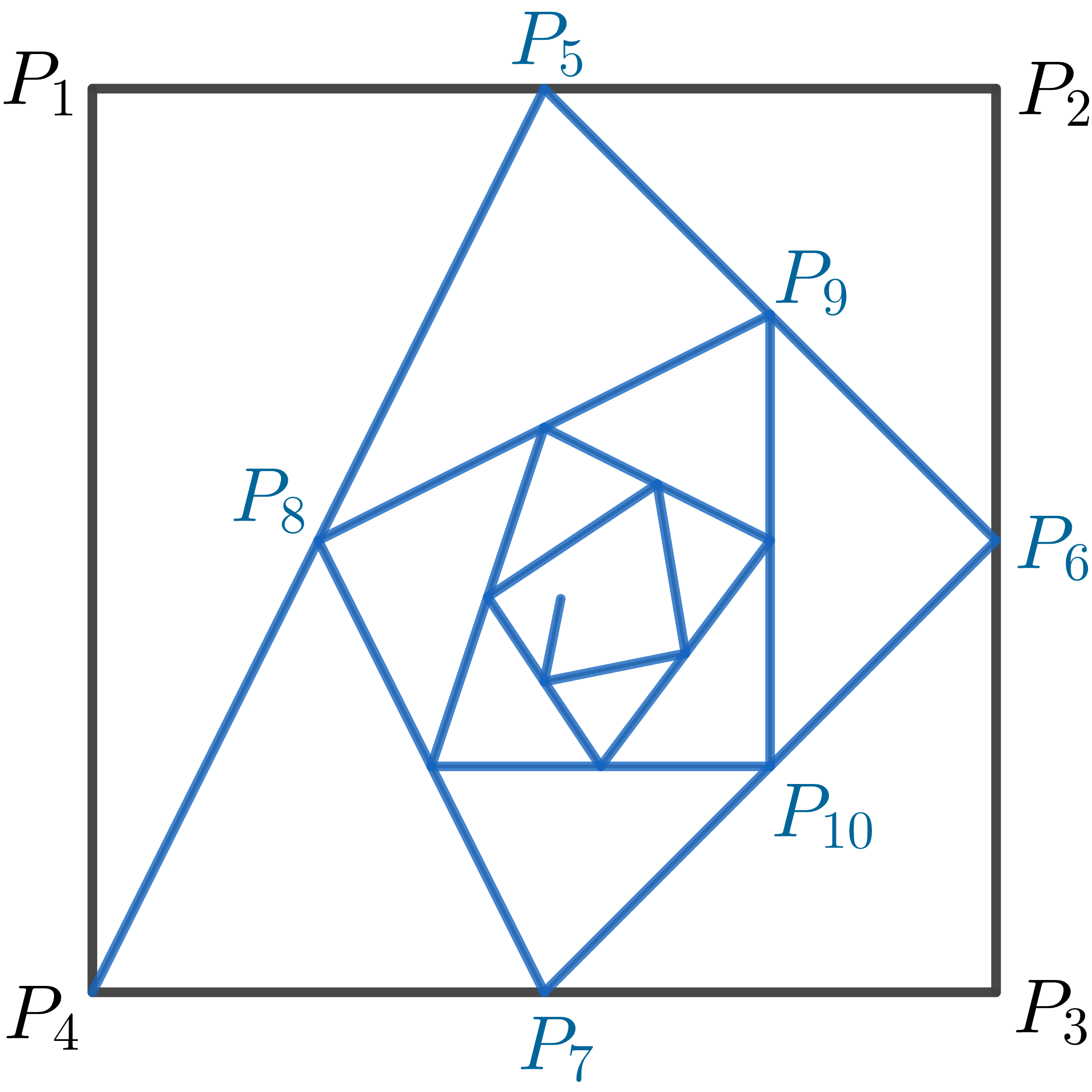# Spiraling Down

Calculus Level 5Starting with the vertices $P_1=(0,1),\ P_2=(1,1),\ P_3=(1,0),\ P_4=(0,0)$ of a square, we construct further points as follows:

• $P_5$ is the midpoint of $P_1P_2$.
• $P_6$ is the midpoint of $P_2P_3$.
• $P_7$ is the midpoint of $P_3P_4,$ and so on.

The spiral path approaches a point $P_\infty=\left(\dfrac{A}{C},\dfrac{B}{C}\right)$ inside the square, where $A, B, C$ are coprime positive integers.

Find $A+B+C.$

×